### Calculate the extension displacement for a linear spring with a certain spring constant and a spring potential energy.

#### Example question:

A linear spring has a potential energy of 25 J and a constant of 510 N/m. How far has it been stretched?

#### Given:

spring potential energy: Us = 25 J

spring constant: k = 510 N/m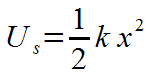Starting formula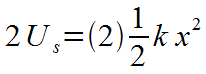Algebra: multiply each side by 2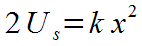Algebra: 2's cancel on right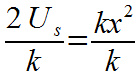Algebra: divide each side by k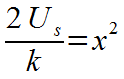Algebra: k's cancel on right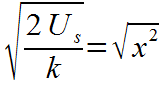Algebra: take square root of each side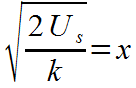Algebra: square root of x^2 is x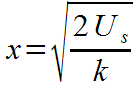Algebra: switch left and right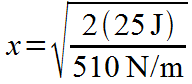Plug in numbers with units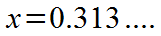Math: sqrt(2(25)/510) = 0.313....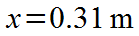Final result with significant figures and unit